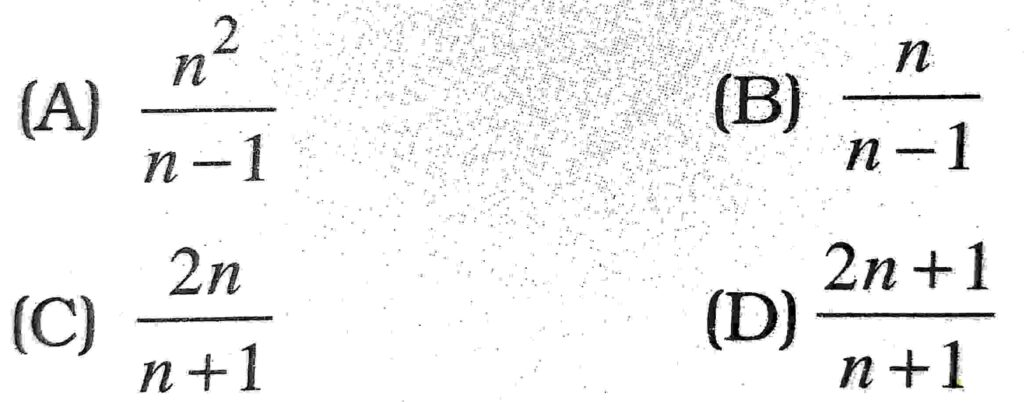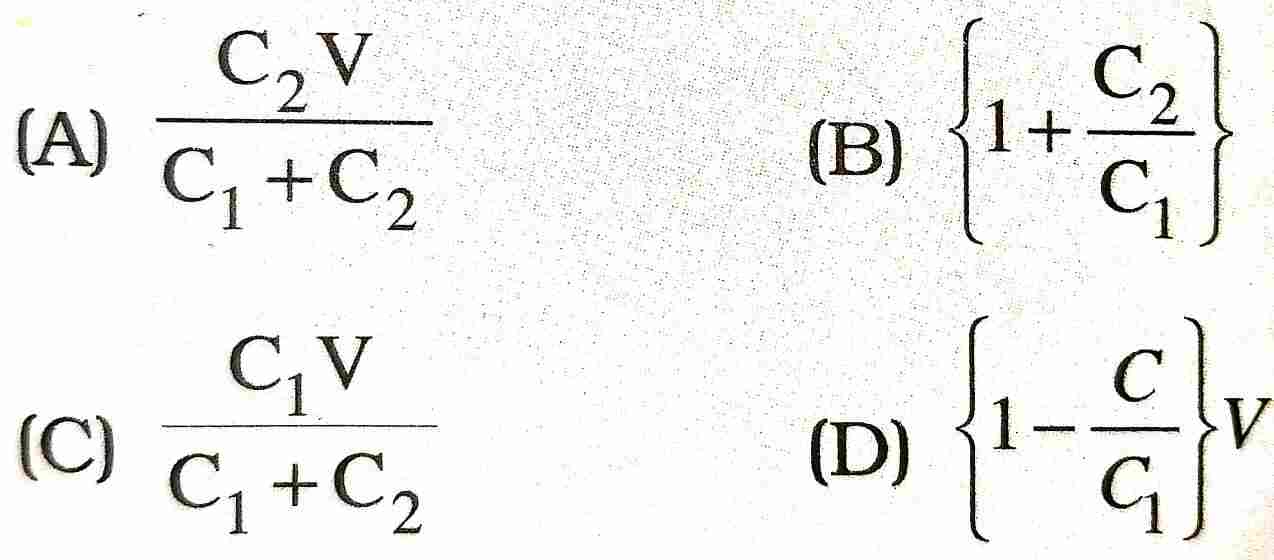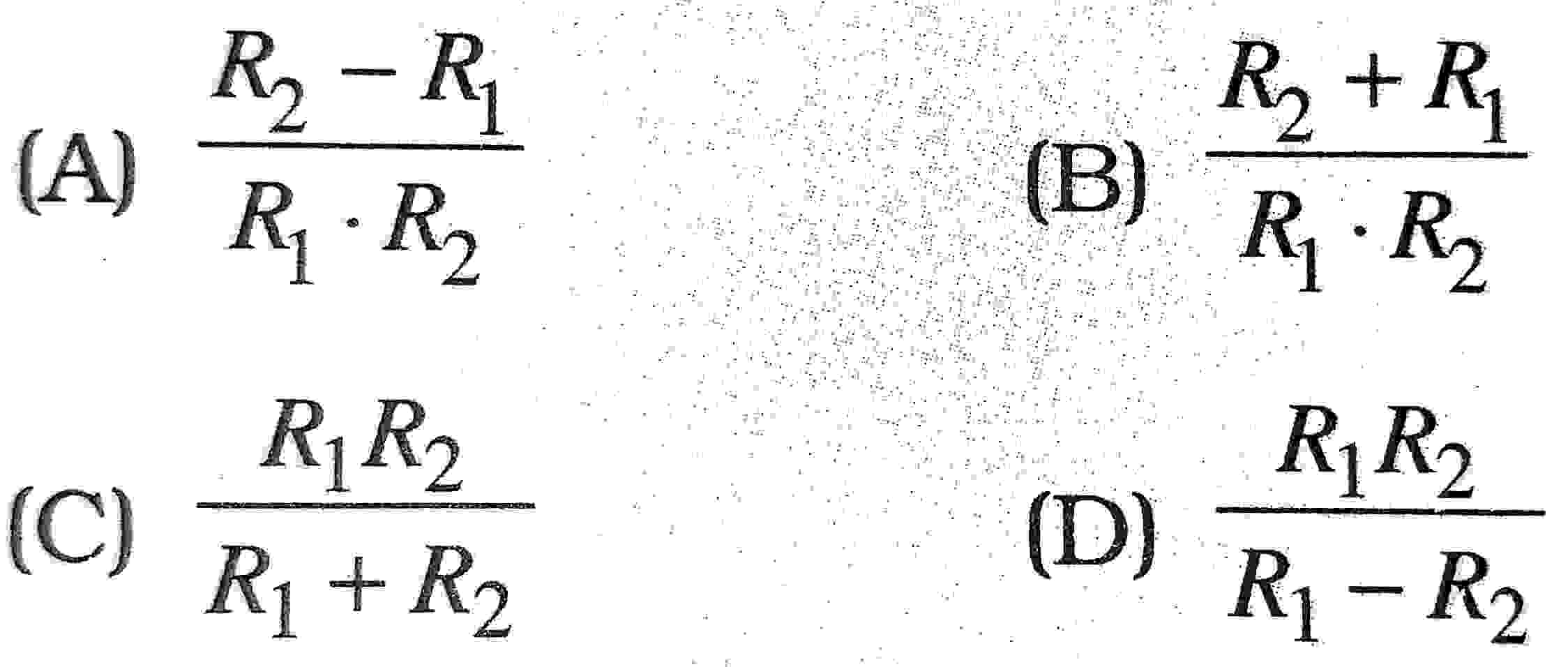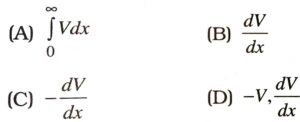1. The dimensions of fall of potential per unit distance are given by :

(A) [MLT-3A-1]
(B) [ML2T-2A-1]
(C) [ML2T-2A-3]
(D) [MLT-2A-2]

2. Which of the following is blocked by a capacitor ?

(A) A.C.
(B) D.C.
(C) Both A.C. and D.C.
(D) Neither A.C. nor D.C.

3. Two copper spheres of the same radius, one solid and the other hollow, are charged to the same potential. Which will have more charge ?

(A) Solid sphere
(B) Hollow sphere
(C) Both will have an equal charge
(D) None of these

4. The capacitance of a capacitor will decrease if we introduce a slab of:

(A) copper
(B) aluminium
(C) zinc
(D) None of these

5. The amount of work required to increase the distance between – 6μC and 4uC from 6 cm to 18 cm will be :

(A) 1.8 J
(B) 2.4 J
(C) 1.8 μ J
(D) 2.4 μ J

6. An electron initially at rest is accelerated through a potential difference of one volt. The energy gained by electron is :

(A) 1 J
(B) 1.6 x 10-19 J
(C) 10-19 J
(D) None of these

7. A bird sitting on a high power line :

(A) gets killed instantly
(B) gets a mild shock
(C) gets a fatal shock
(D) is not affected practically

8. Choose the SI unit of electric potentia energy :

(A) Joule
(B) Coulomb
(C) Netwon per coulomb
(D) Erg

9. The capacitance of earth, viewed as spherical conductor of radius 6408 km is.

(A) 1420 μF
(B) 712 μF
(C) 680 μF
(D) 540 μF

10. A capacitor is connected to a cell of emin having some internal resistance r, the potential across the :

(A) cell is < E
(B) cell is E
(C) capacitor is > E
(D) capacitor is E

11. If a +ve charge is moved from low to high potential region, the electric potentiall energy :

(A) decreases
(B) increases
(C) remain the same
(D) may increase or decrease

12. Minimum number of capacitor of 2μF each required to obtain a capacitance of 5μF will be :

(A) 4
(B) 3
(C) 5
(D) 6

13. When a dielectric slab is introduced between the plates of a parallel plate capacitor which remains connected to a battery then charge on the plates relative to earlier charge is :

(A) more
(B) less
(C) less or may be more
(D) same

14. Choose the vector physical quantity :

(A) Electric flux
(B) Electric potential
(C) Electric potential energy
(D) Electric intensity

15. The surface density of charge is measure :

(A) Cm-1
(B) Cm-2
(C) Cm-3
(D) JC-1

16. A polythene piece rubbed with wool is found to have a negative charge of 6.4 x 10-9c. How many electrons have been transferred during the process ?

(A) 4 x 1012
(B) 4 x 1011
(C) 4 x 1010
(D) None of these

17. In the above question :

(A) no electrons are transferred
(B) electrons are transferred from wool to x polythene
(C) electrons are transferred from polythene to wool
(D) nothing can be decided

18. In previous question, mass transferred to polythene is :

(A) 3.64 x 10-20 kg
(B) 4 x 10-28 kg
(C) 4 x 1010 kg
(D) None of these

19. What is the number of electrons in 100 g water ?

(A) 3.35 x 1024
(B) 3.35 x 1025
(C) 6.02 x 1023
(D) None of these

20. A uniform electric field of 10 NC-1 exists in the vertically downward direction. What is the increase in the electric potential as one goes up through a height of 300 cm ?

(A) 15 V
(B) 30 V
(C) 45 V
(D) None of these

21. The electric potential of earth is taken as :

(A) zero
(B) infinity
(C) unity
(D) None of these

22. The capacity of an isolated sphere is increases n times when it is enclosed by an earth concentric sphere. The ratio of their radii is :23. The capacity of an isolated conducting sphere of radius R is proportional to :

(A) R2
(B) 1/R2
(C) 1/R
(D) R

24. The value of a charge q at the centre of two equal and like charges Q so that the three charges are in equilibrium is :

(A) Q
(B) Q/2
(C) Q/4
(D) -Q/4

25. Coulomb’s law is given by F= Kq1q2rn, where n is :

(A) 1/2
(B) -2
(C) 2
(D) -1

26. When dielectric is placed into the gap of parallel plate capacitor, the capacitance of capacitor ?

(A) decreases
(B) increases
(C) remains same
(D) None of these

27. What is the net charge on a charged capacitor ?

(A) Zero
(B) 1μC
(C) 10
(D) Infinity

28. A capacitor of capacity C1 is charged upto V volts and then connected to an uncharged capacitor of capacity C2. Then final potential difference across each will be :29. Conducting sphere of radius R1 is covered by concentric sphere of radius R2. Capacity of this combination is proportional to :30. Let V be the electric potential at a given point, then the electric field Fx along x- direction at that point is given by :31. The electric potential due to a small electric dipole at a large distance from the centre of the dipole is proportional to :

(A) r
(D) 1/r
(C) 1/r2
(D) 1/r3

32. A series combination of there capacitors of capacitance lμF, 2μF and 8μF is connected in series to a battery of emf 13 Volt. The potential difference across the plates of 2μF capacitor will be :

(A) IV
(B) 8V
(C) 13/3 V
(D) 4V